# PID VI

## LabVIEW 2018 FPGA Module Help

Edition Date: March 2018
Part Number: 371599P-01
View Product InfoLabVIEW 2016 FPGA Module HelpLabVIEW 2017 FPGA Module HelpLabVIEW 2018 FPGA Module HelpLabVIEW 2019 FPGA Module HelpLabVIEW 2020 FPGA Module Help

Owning Palette: Control VIs

Requires: FPGA Module

Implements a single-precision floating-point PID algorithm for PID applications with high-speed control and/or high channel count on an FPGA target. You can use this VI to create single-channel, multi-channel, and multi-rate control applications.

The PID algorithm features control action range and uses an integrator anti-windup calculation to limit the effect of the integral action during transients. The PID algorithm also features bumpless controller output for PID gain changes.

Use the pull-down menu to select an instance of this VI.

 Select an instance PID (PID)PID (PI)PID (PD)

## PID (PID)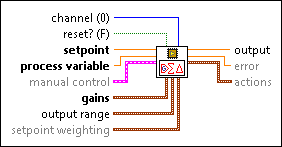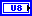channel specifies the channel that this VI processes. The valid value range is [0, 255]. The default is 0.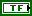reset? specifies whether to reset the internal state of the channel that you specify. The default is FALSE.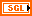setpoint specifies the value that you want process variable to attain. In equations that define the PID controller, SP represents setpoint.process variable specifies the value of the variable that you want to control. In equations that define the PID controller, PV represents process variable.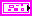manual control specifies the control mode and the control output value for the manual control mode.manual? specifies whether to use the automatic or manual control mode. The default is FALSE, which specifies to use the automatic control mode.manual input specifies the control output value for the manual control mode. The default is 0.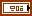gains specifies the proportional gain, integral gain, derivative gain, and filter coefficient parameters of the controller. The proportional gain, integral gain, and derivative gain are normalized. Use the Convert PID Gains VI to convert PID gains into the form that gains requires.proportional gain specifies the normalized proportional gain of the controller. In equations that define the PID controller, Kp represents proportional gain.integral gain specifies the normalized integral gain of the controller. In equations that define the PID controller, Ki represents integral gain.derivative gain specifies the normalized derivative gain of the controller. In equations that define the PID controller, Kd represents derivative gain.filter coefficient specifies the derivative lowpass filter coefficient of the controller. The valid value range is [0, 1]. Increasing the value of filter coefficient increases damping of the derivative action. In equations that define the PID controller, a represents filter coefficient.output range specifies the range for the control output value. If the control output value is outside output range, this VI coerces the value to fall within the range and returns the coerced value as the control output value. This VI implements integrator anti-windup when the control output is saturated at the specified minimum or maximum value.output high specifies the maximum control output value. The default is Inf.output low specifies the minimum control output value. The default is -Inf.setpoint weighting specifies corrections to apply to the error values of the controller. setpoint weighting tunes the proportional action and the derivative action.proportional weighting specifies the relative emphasis of setpoint tracking to disturbance rejection. The valid value range is [0, 1]. The default is 1, which is appropriate for most applications. In equations that define the PID controller, beta represents proportional weighting.derivative weighting specifies an amount by which to weight the error applied to the derivative action. The default value is 0, which avoids the derivative kick. The valid value range is [0, 1]. In equations that define the PID controller, gamma represents derivative weighting.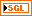output returns the control output value of the PID algorithm.error returns the difference between setpoint and process variable.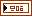actions returns the values of the proportional action, the integral action, and the derivative action in the PID algorithm.proportional action returns the value of the proportional action.integral action returns the value of the integral action.derivative action returns the value of the derivative action.

## PID (PI)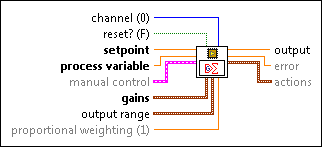channel specifies the channel that this VI processes. The valid value range is [0, 255]. The default is 0.reset? specifies whether to reset the internal state of the channel that you specify. The default is FALSE.setpoint specifies the value that you want process variable to attain. In equations that define the PID controller, SP represents setpoint.process variable specifies the value of the variable that you want to control. In equations that define the PID controller, PV represents process variable.manual control specifies the control mode and the control output value for the manual control mode.manual? specifies whether to use the automatic or manual control mode. The default is FALSE, which specifies to use the automatic control mode.manual input specifies the control output value for the manual control mode. The default is 0.gains specifies the PI gain parameters. The gains are without units.proportional gain specifies the normalized proportional gain of the controller. In equations that define the PID controller, Kp represents proportional gain.integral gain specifies the normalized integral gain of the controller. In equations that define the PID controller, Ki represents integral gain.output range specifies the range for the control output value. If the control output value is outside output range, this VI coerces the value to fall within the range and returns the coerced value as the control output value. This VI implements integrator anti-windup when the control output is saturated at the specified minimum or maximum value.output high specifies the maximum control output value. The default is Inf.output low specifies the minimum control output value. The default is -Inf.proportional weighting specifies the relative emphasis of setpoint tracking to disturbance rejection. The valid value range is [0, 1]. The default is 1, which is appropriate for most applications. In equations that define the PID controller, beta represents proportional weighting.output returns the control output value of the PID algorithm.error returns the difference between setpoint and process variable.actions returns the values of the proportional action and the integral action in the PI algorithm.proportional action returns the value of the proportional action.integral action returns the value of the integral action.

## PID (PD)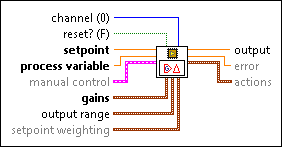channel specifies the channel that this VI processes. The valid value range is [0, 255]. The default is 0.reset? specifies whether to reset the internal state of the channel that you specify. The default is FALSE.setpoint specifies the value that you want process variable to attain. In equations that define the PID controller, SP represents setpoint.process variable specifies the value of the variable that you want to control. In equations that define the PID controller, PV represents process variable.manual control specifies the control mode and the control output value for the manual control mode.manual? specifies whether to use the automatic or manual control mode. The default is FALSE, which specifies to use the automatic control mode.manual input specifies the control output value for the manual control mode. The default is 0.gains specifies the PD gain parameters. The gains are without units.proportional gain specifies the normalized proportional gain of the controller. In equations that define the PID controller, Kp represents proportional gain.derivative gain specifies the normalized derivative gain of the controller. In equations that define the PID controller, Kd represents derivative gain.filter coefficient specifies the derivative lowpass filter coefficient of the controller. The valid value range is [0, 1]. Increasing the value of filter coefficient increases damping of the derivative action. In equations that define the PID controller, a represents filter coefficient.output range specifies the range for the control output value. If the control output value is outside output range, this VI coerces the value to fall within the range and returns the coerced value as the control output value. This VI implements integrator anti-windup when the control output is saturated at the specified minimum or maximum value.output high specifies the maximum control output value. The default is Inf.output low specifies the minimum control output value. The default is -Inf.setpoint weighting specifies a set of weighting that adjust the error applied to the proportional action and the derivative action.proportional weighting specifies the relative emphasis of setpoint tracking to disturbance rejection. The valid value range is [0, 1]. The default is 1, which is appropriate for most applications. In equations that define the PID controller, beta represents proportional weighting.derivative weighting specifies an amount by which to weight the error applied to the derivative action. The default value is 0, which avoids the derivative kick. The valid value range is [0, 1]. In equations that define the PID controller, gamma represents derivative weighting.output returns the control output value of the PID algorithm.error returns the difference between setpoint and process variable.actions returns the values of the proportional action and the derivative action in the PD algorithm.proportional action returns the value of the proportional action.derivative action returns the value of the derivative action.

## PID Details

The PID VI calculates the output, u(k), according to the following equations: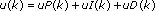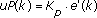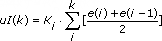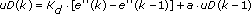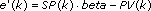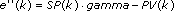where

Kp is proportional gain

Ki is integral gain

Kd is derivative gain

a is filter coefficient

SP is setpoint

beta is proportional weighting

PV is process variable

gamma is derivative weighting

## Examples

Refer to the following VIs for examples of using the PID VI:

• labview\examples\CompactRIO\FPGA Fundamentals\FPGA Math and Analysis\Floating-point PID\Multi-Channel PID\Multi-Channel PID.lvproj
• labview\examples\CompactRIO\FPGA Fundamentals\FPGA Math and Analysis\Floating-point PID\Multi-Rate PID\Multi-Rate PID.lvproj
• labview\examples\R Series\FPGA Fundamentals\FPGA Math and Analysis\Floating-point PID\Multi-Channel PID\Multi-Channel PID.lvproj
• labview\examples\R Series\FPGA Fundamentals\FPGA Math and Analysis\Floating-point PID\Multi-Rate PID\Multi-Rate PID.lvproj